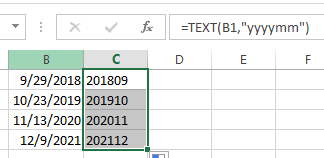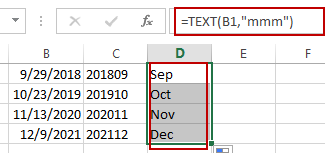# Extract Month and Year from Date in Excel

This post will guide you how to extract month and year from a date in excel. How do I get month and year from date cells  with a formula in Excel. How to convert date to month and year in Excel.

## Extract Month and Year from Date

If you want to extract month and year from a date in Cell, you can use a formula based on the TEXT function.
Assuming that you have a list of data in range B1:B4 that contain dates, and you want to convert the normal excel date into year and month format (yyyymm), How to achieve it. You can use the following format:

`=TEXT(B1,"yyyymm")`

Type this formula into a blank cell and then press Enter key in your keyboard. and drag the AutoFill Handle over other cells to apply this formul.

###This formula will get a date with the year and month only from the orignial date in Cells.

If you only want to extract month from a date, and you can use the following formula:

`=TEXT(B1,"mmm")`

###If you want to convert date to year format only, you can use the following formula:

`=TEXT(B1,"yyyy")`

### Related Functions

• Excel Text function
The Excel TEXT function converts a numeric value into text string with a specified format. The TEXT function is a build-in function in Microsoft Excel and it is categorized as a Text Function. The syntax of the TEXT function is as below: = TEXT (value, Format code)…